# Test: Time Varying EMT Fields

## 10 Questions MCQ Test Topicwise Question Bank for Electronics Engineering | Test: Time Varying EMT Fields

Description
Attempt Test: Time Varying EMT Fields | 10 questions in 30 minutes | Mock test for Electrical Engineering (EE) preparation | Free important questions MCQ to study Topicwise Question Bank for Electronics Engineering for Electrical Engineering (EE) Exam | Download free PDF with solutions
QUESTION: 1

### If the magnetic field vector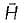has only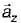component given by Hz = 3x cosβ+ 6y sinγ and if the field is invariant with time, what is the expression for current density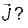Solution:

Since,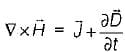and as the field is invariant, therefore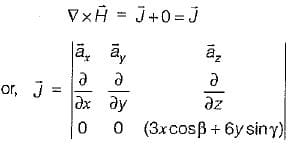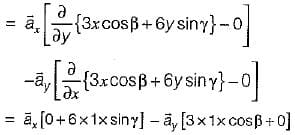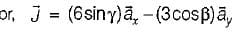QUESTION: 2

### The conduction current in a straight copper wire of cross-sectional area A = 1.5 x 10-5 m2 is I = 2 A. If the constitutive parameters of copper are μ = μ0 = 4π x 10-7 H/m,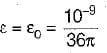F/m and σ = 5.8 x 107 mho/m, then the displacement current Id in the wire at a frequency of f = 1 GHz would be

Solution:

Let the copper wire lies along the z-axis of the cylindrical coordinate system. The magnetic field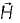is then directed in azimuthal direction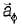and electric field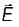is directed in the z-direction.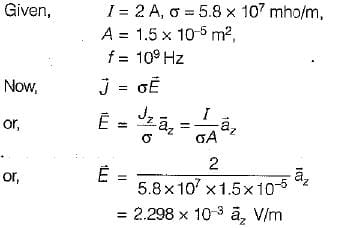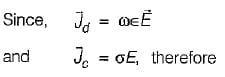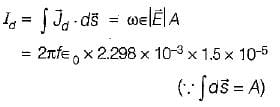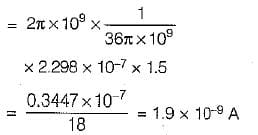or, Id = 1.9 nA

QUESTION: 3

### A rectangular loop of length a = 1 meter and width b = 80 cm is placed in a uniform magnetic field. What is the maximum value of induced emf if the magnetic flux density B = 0.1 Wb/m2 is constant and the loop rotates about the x-axis with a frequency of 50 Hz?

Solution:

The induced emf in uniform magnetic field is given by
v = ω(ab) B sin ωt
or, v = ωAB sin ωt
(where, A = ab = Area of rectangular loop)
or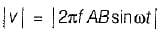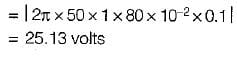QUESTION: 4

A straight conducting wire of length 50 cm is moved in a direction at right angles to its length in a region in air permitted by a uniform flux density B of magnitude B = 1 Wb/m2. If the magnetic flux density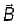is perpendicular to both the direction of the motion and the length of the wire and the magnitude of the velocity of motion \/ = 10 m/s, the induced voltage in the conductor would be

Solution:

Induced emf in the conductor is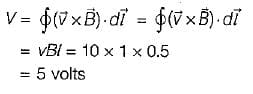QUESTION: 5

A Faraday disc of 10 cm radius rotates at 5000 rpm in a uniform magnetic field of 250 mWb/m2, the field being normal to the plane of the disc. What is the emf between rpm and axis assuming the diameter of the axis very small?

Solution: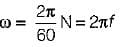where, N = Number of revolutions/min
∴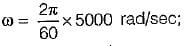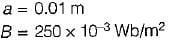The emf induced is,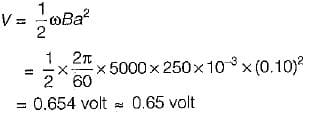QUESTION: 6

What is the emf developed about the path r = 0.5, z = 0 and at t = 0, if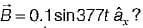Solution:

Given B = 0.1 Wb/m2
r = 0.5 m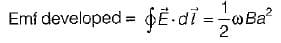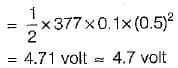QUESTION: 7

A square loop of wire 25 cm has a voltmeter (of infinite impedance) connected in series with one side. The plane of the loop is perpendicular to the magnetic field and the frequency is 10 MHz. If the maximum intensity is 1 Amp/m, then the voltage indicated by the meter when the loop is placed in the alternating field would be

Solution: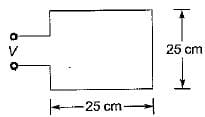Given,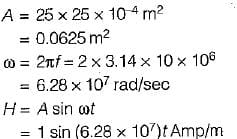The required induced voltage is given by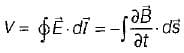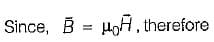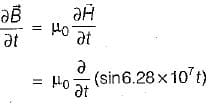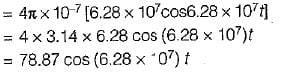Hence, the maximum induced voltage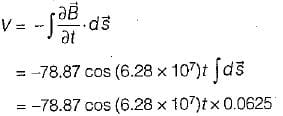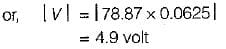QUESTION: 8

Assertion (A): The total e.m.f. induced in a circuit is equal to the time rate of decrease of the total magnetic flux linking the circuit.
Reason (R): Changing magnetic field will induce on electric field.

Solution:

Both assertion and reason are individually correct statements.
Since induced emf,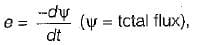thus due to negative sign, the total emf induced is equal to the time rate of decrease of the total magnetic flux linking the circuit. (The negative sign supports the Lenz’s law).

QUESTION: 9

Assertion (A): Motional induction or flux cutting law gives the e.m.f. induced in a moving conductor w.r.t observer in a magnetic field.
Reason (R): The motional emf equation depends on the velocity of the conductor and its position.

Solution:
• Assertion (A) is a correct statement since the motional induction or flux cutting law are given by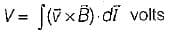or, V = vBl volts
• Motional emf equation depends only on the velocity of the conductor and not its position. Thus, reason (R) is not a correct statement,
QUESTION: 10

In a material for which conductivity σ = 5 Siemen/m and ∈r = 1, the electric field intensity is E = 250 sin 1010 t V/m. What is the frequency at which the displacement and conduction current densities will be equal?

Solution: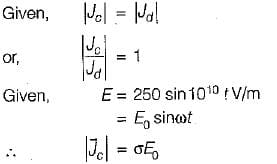= Conduction current density
and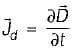= Displacement current density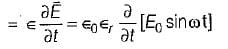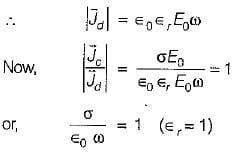or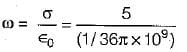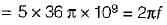or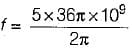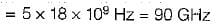Use Code STAYHOME200 and get INR 200 additional OFF Use Coupon Code